Updating search results...

# 18 Results

View
Selected filters:
• MCCRS.Math.Content.HSA-REI.C.6Unrestricted Use
CC BY
Rating
0.0 stars

The given solutions for this task involve the creation and solving of a system of two equations and two unknowns, with the caveat that the context of the problem implies that we are interested only in non-negative integer solutions. Indeed, in the first solution, we must also restrict our attention to the case that one of the variables is further even.

Subject:
Mathematics
Algebra
Material Type:
Activity/Lab
Provider:
Illustrative Mathematics
Provider Set:
Illustrative Mathematics
Author:
Illustrative Mathematics
Date Added:
01/18/2013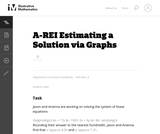Unrestricted Use
CC BY
Rating
0.0 stars

This is a task from the Illustrative Mathematics website that is one part of a complete illustration of the standard to which it is aligned. Each task has at least one solution and some commentary that addresses important asects of the task and its potential use. Here are the first few lines of the commentary for this task: Jason and Arianna are working on solving the equations \begin{align} 6x + 17y &= 100\\ 5x + 9y &= 86. \end{align} Rounding their answer to the nearest ...

Subject:
Mathematics
Material Type:
Activity/Lab
Provider:
Illustrative Mathematics
Provider Set:
Illustrative Mathematics
Author:
Illustrative Mathematics
Date Added:
03/20/2014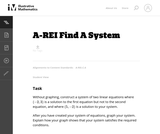Unrestricted Use
CC BY
Rating
0.0 stars

This is a task from the Illustrative Mathematics website that is one part of a complete illustration of the standard to which it is aligned. Each task has at least one solution and some commentary that addresses important asects of the task and its potential use. Here are the first few lines of the commentary for this task: Without graphing, construct a system of two linear equations where $(-2,3)$ is a solution to the first equation but not to the second equation, and whe...

Subject:
Mathematics
Material Type:
Activity/Lab
Provider:
Illustrative Mathematics
Provider Set:
Illustrative Mathematics
Author:
Illustrative Mathematics
Date Added:
05/26/2013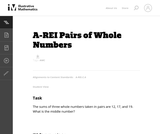Unrestricted Use
CC BY
Rating
0.0 stars

This is a task from the Illustrative Mathematics website that is one part of a complete illustration of the standard to which it is aligned. Each task has at least one solution and some commentary that addresses important asects of the task and its potential use. Here are the first few lines of the commentary for this task: The sums of three whole numbers taken in pairs are 12, 17, and 19. What is the middle number?...

Subject:
Mathematics
Material Type:
Activity/Lab
Provider:
Illustrative Mathematics
Provider Set:
Illustrative Mathematics
Author:
Illustrative Mathematics
Date Added:
03/27/2013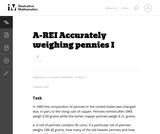Unrestricted Use
CC BY
Rating
4.0 stars

This problem involves solving a system of algebraic equations from a context: depending how the problem is interpreted, there may be one equation or two.

Subject:
Mathematics
Algebra
Material Type:
Activity/Lab
Provider:
Illustrative Mathematics
Provider Set:
Illustrative Mathematics
Author:
Illustrative Mathematics
Date Added:
08/15/2012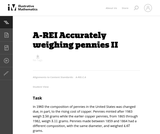Unrestricted Use
CC BY
Rating
0.0 stars

This task is a somewhat more complicated version of "Accurately weighing pennies I'' as a third equation is needed in order to solve part (a) explicitly. Instead, students have to combine the algebraic techniques with some additional problem-solving (numerical reasoning, informed guess-and-check, etc.)

Subject:
Mathematics
Algebra
Material Type:
Activity/Lab
Provider:
Illustrative Mathematics
Provider Set:
Illustrative Mathematics
Author:
Illustrative Mathematics
Date Added:
08/15/2012Conditional Remix & Share Permitted
CC BY-NC-SA
Rating
0.0 stars

Students connect polynomial arithmetic to computations with whole numbers and integers.  Students learn that the arithmetic of rational expressions is governed by the same rules as the arithmetic of rational numbers.  This unit helps students see connections between solutions to polynomial equations, zeros of polynomials, and graphs of polynomial functions.  Polynomial equations are solved over the set of complex numbers, leading to a beginning understanding of the fundamental theorem of algebra.  Application and modeling problems connect multiple representations and include both real world and purely mathematical situations.

Subject:
Algebra
Material Type:
Module
Provider:
New York State Education Department
Provider Set:
EngageNY
Date Added:
05/14/2013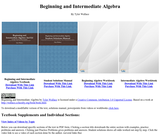Unrestricted Use
CC BY
Rating
4.0 stars

Beginning and Intermediate Algebra by Tyler Wallace is a textbook licensed under a Creative Commons Attribution 3.0 Unported License. There is also a student guide and supplemental videos for each section.

Subject:
Algebra
Material Type:
Textbook
Provider:
Big Bend Community College
Author:
Tyler Wallace
Date Added:
01/29/2014Only Sharing Permitted
CC BY-NC-ND
Rating
0.0 stars

This lesson unit is intended to help teachers assess how well students are able to use linear inequalities to create a set of solutions. In particular, the lesson will help teachers identify and assist students who have difficulties in: representing a constraint by shading the correct side of the inequality line; and understanding how combining inequalities affects a solution space.

Subject:
Mathematics
Material Type:
Assessment
Lesson Plan
Provider:
Shell Center for Mathematical Education
Provider Set:
Mathematics Assessment Project (MAP)
Date Added:
04/26/2013Read the Fine Print
Educational Use
Rating
0.0 stars

Students explore material properties by applying some basic principles of heat transfer. They use calorimeters to determine the specific heat of three substances: aluminum, copper and another of their choice. Each substance is cooled in a freezer and then placed in the calorimeter. The temperature change of the water and the substance are used in heat transfer equations to determine the specific heat of each substance. The students compare their calculated values with tabulated data.

Subject:
Engineering
Physics
Material Type:
Activity/Lab
Provider:
TeachEngineering
Provider Set:
TeachEngineering
Author:
James Prager
Janet Yowell
Malinda Zarske
Megan Schroeder
Date Added:
09/18/2014Conditional Remix & Share Permitted
CC BY-NC
Rating
0.0 stars

In this activity, students write and solve a system of two linear equations to explore the numerical and graphical meaning of "solution." The activity closes by asking students to apply what they've learned to similar situations.

Subject:
Mathematics
Algebra
Material Type:
Lesson Plan
Author:
Shawn Dykstra
Date Added:
11/14/2017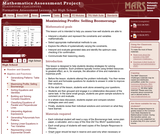Only Sharing Permitted
CC BY-NC-ND
Rating
0.0 stars

This lesson unit is intended to help teachers assess how well students are able to: interpret a situation and represent the constraints and variables mathematically; select appropriate mathematical methods to use; explore the effects of systematically varying the constraints; interpret and evaluate the data generated and identify the optimum case, checking it for confirmation; and communicate their reasoning clearly.

Subject:
Measurement and Data
Material Type:
Assessment
Lesson Plan
Provider:
Shell Center for Mathematical Education
Provider Set:
Mathematics Assessment Project (MAP)
Date Added:
04/26/2013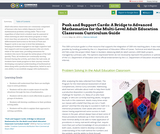Conditional Remix & Share Permitted
CC BY-NC-SA
Rating
5.0 stars

Adult education classrooms are commonly comprised of learners who have widely disparate levels of mathematical problem-solving skills. This is true regardless of what level a student may be assessed at when entering an adult education program or what level class they are placed in. Providing students with differentiated instruction in the form of Push and Support cards is one way to level this imbalance, keeping all students engaged in one high-cognitive task that supports and encourages learners who are stuck, while at the same time, providing extensions for students who move through the initial phase of the task quickly. Thus, all
students are continually moving forward during the activity, and when the task ends, all students have made progress in their journey towards developing conceptual understanding of mathematical ideas along with a productive disposition, belief in one’s own ability to successfully engage with mathematics.

Subject:
Mathematics
Material Type:
Lesson Plan
Module
Teaching/Learning Strategy
Author:
Patricia Helmuth
Date Added:
05/24/2018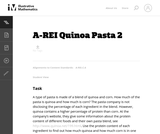Unrestricted Use
CC BY
Rating
0.0 stars

This task has some aspects of a mathematical modeling problem (SMP 4) and it also illustrates SMP 1 (Making sense of a problem). Students are given all the relevant information on the nutritional labels, but they have to figure out how to use this information. They have to come up with the idea that they can set up two equations in two unknowns to solve the problem.

Subject:
Mathematics
Algebra
Material Type:
Activity/Lab
Provider:
Illustrative Mathematics
Provider Set:
Illustrative Mathematics
Author:
Illustrative Mathematics
Date Added:
09/13/2012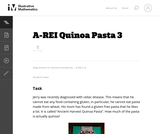Unrestricted Use
CC BY
Rating
0.0 stars

This tasks is an example of a mathematical modeling problem (SMP 4) and it also illustrates SMP 1 (Making sense of a problem). Students are only told that there are two ingredients in the pasta and they have a picture of the box. It might even be better to just show the picture of the box, or to bring in the box and ask the students to pose the question themselves.

Subject:
Mathematics
Algebra
Material Type:
Activity/Lab
Provider:
Illustrative Mathematics
Provider Set:
Illustrative Mathematics
Author:
Illustrative Mathematics
Date Added:
09/13/2012
Remix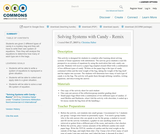Conditional Remix & Share Permitted
CC BY-NC
Rating
0.0 stars

Students are given 2 different types of candy in a mystery bag and they will have to write their own system of equations. Then they will analyze the equations to determine how many of each type are in the bags.

Subject:
Mathematics
Material Type:
Activity/Lab
Author:
Christina Haber
Date Added:
08/08/2019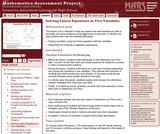Only Sharing Permitted
CC BY-NC-ND
Rating
0.0 stars

This lesson unit is intended to help teachers assess how well students are able to formulate and solve problems using algebra and, in particular, to identify and help students who have the following difficulties: solving a problem using two linear equations with two variables; and interpreting the meaning of algebraic expressions.

Subject:
Algebra
Material Type:
Assessment
Lesson Plan
Provider:
Shell Center for Mathematical Education
Provider Set:
Mathematics Assessment Project (MAP)
Date Added:
04/26/2013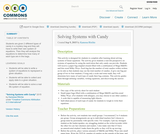Unrestricted Use
CC BY
Rating
5.0 stars

Students are given 2 different types of candy in a mystery bag and they will have to write their own system of equations. Then they will analyze the equations to determine how many of each type are in the bags.

Subject:
Mathematics
Material Type:
Activity/Lab
Author:
Kanessa Ritchie
Date Added:
01/28/2016